Comparison
Comparison close
Results

CCQM-K3 was placed in the KCDB archives on 18 September 2023

CCQM-K3, EUROMET.QM-K3, APMP.QM-K3 and COOMET.QM-K3

MEASURAND : Amount-of-substance fraction of automotive emission gases

NOMINAL VALUES for Carbon monoxide : ~ 30 mmol/mol

Degrees of equivalence Di and expanded uncertainty Ui (k = 2) expressed in mmol/mol

Please click onto the graph to get an enlarged version.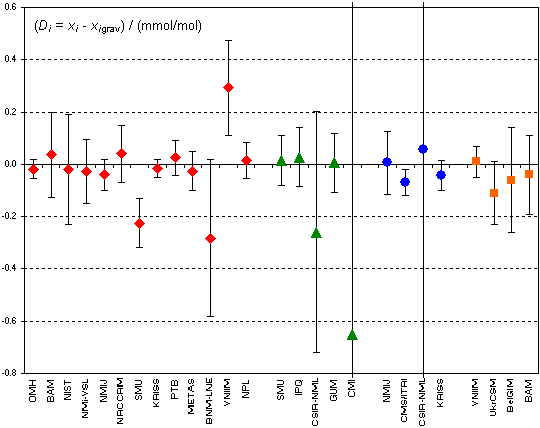Red diamonds: participants in CCQM-K3
Green triangles: participants in EUROMET.QM-K3
Blue circles: participants in APMP.QM-K3

Orange squares: participants in COOMET.QM-K3

CCQM-K3 was placed in the KCDB archives on 18 September 2023

CCQM-K3, EUROMET.QM-K3, APMP.QM-K3 and COOMET.QM-K3

MEASURAND : Amount-of-substance fraction of automotive emission gases

NOMINAL VALUES for Carbon dioxide : ~ 135 mmol/mol

Degrees of equivalence Di and expanded uncertainty Ui (k = 2) expressed in mmol/mol

Please click onto the graph to get an enlarged version.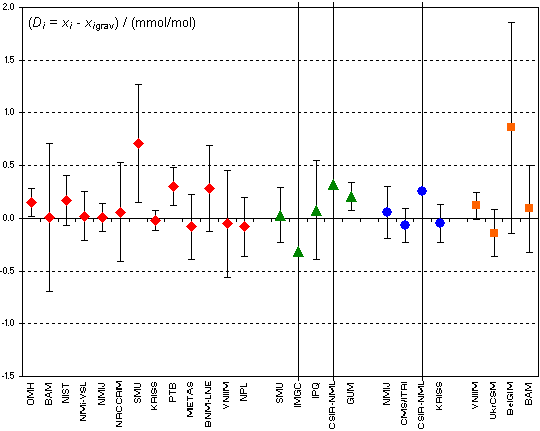Red diamonds: participants in CCQM-K3
Green triangles: participants in EUROMET.QM-K3
Blue circles: participants in APMP.QM-K3

Orange squares: participants in COOMET.QM-K3

CCQM-K3 was placed in the KCDB archives on 18 September 2023

CCQM-K3, EUROMET.QM-K3, APMP.QM-K3 and COOMET.QM-K3

MEASURAND : Amount-of-substance fraction of automotive emission gases

NOMINAL VALUES for Propane : ~ 2 mmol/mol

Degrees of equivalence Di and expanded uncertainty Ui (k = 2) expressed in mmol/mol

Please click onto the graph to get an enlarged version.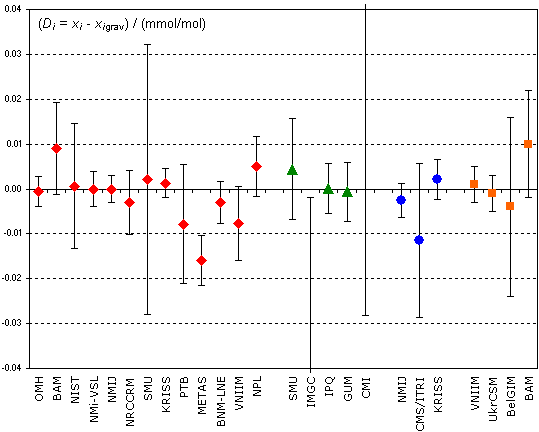Red diamonds: participants in CCQM-K3
Green triangles: participants in EUROMET.QM-K3
Blue circles: participants in APMP.QM-K3

Orange squares: participants in COOMET.QM-K3

Comparison
Comparison close
Results

CCQM-K3 was placed in the KCDB archives on 18 September 2023

CCQM-K3, EUROMET.QM-K3, APMP.QM-K3 and COOMET.QM-K3

MEASURAND : Amount-of-substance fraction of automotive emission gases

NOMINAL VALUES for Carbon monoxide : ~ 30 mmol/mol

 Lab i Di Ui /(mmol/mol) /(mmol/mol) OMH -0.018 0.038 BAM 0.037 0.162 NIST -0.020 0.209 NMi-VSL -0.028 0.122 NMIJ -0.040 0.059 NRCCRM 0.040 0.109 SMU -0.225 0.094 KRISS -0.015 0.034 PTB 0.026 0.067 METAS -0.026 0.074 BNM-LNE -0.282 0.301 VNIIM 0.293 0.181 NPL 0.015 0.068 SMU 0.014 0.096 IPQ 0.027 0.112 CSIR-NML -0.260 0.461 GUM 0.005 0.112 CMI -0.652 4.400 NMIJ 0.01 0.12 CMS/ITRI -0.07 0.05 CSIR-NML 0.06 1.60 KRISS -0.04 0.06 VNIIM 0.01 0.06 UkrCSM -0.11 0.12 BelGIM -0.06 0.20 BAM -0.04 0.15

In dark blue: laboratories participant in CCQM-K3
In green
: laboratories participant in EUROMET.QM-K3 (indicated as * in "Lab j" list)
In blue
: laboratories participant in APMP.QM-K3
In orange: laboratories participant in COOMET.QM-K3

Additional data: pair-wise degrees of equivalence inside APMP.QM-K3 and COOMET.QM-K3: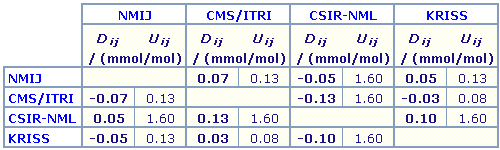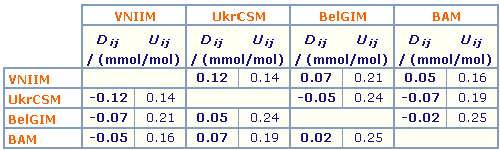All results, including relative degrees of equivalence for COOMET participants, are presented under A4 printable format in Summary Results (.PDF file).

CCQM-K3 was placed in the KCDB archives on 18 September 2023

CCQM-K3, EUROMET.QM-K3, APMP.QM-K3 and COOMET.QM-K3

MEASURAND : Amount-of-substance fraction of automotive emission gases

NOMINAL VALUES for Carbon dioxide : ~ 135 mmol/mol

 Lab i Di Ui /(mmol/mol) /(mmol/mol) OMH 0.15 0.14 BAM 0.01 0.70 NIST 0.17 0.24 NMi-VSL 0.02 0.23 NMIJ 0.01 0.13 NRCCRM 0.06 0.47 SMU 0.71 0.56 KRISS -0.02 0.09 PTB 0.30 0.18 METAS -0.08 0.31 BNM-LNE 0.28 0.41 VNIIM -0.05 0.51 NPL -0.08 0.28 SMU 0.03 0.26 IMGC -0.32 5.10 IPQ 0.08 0.47 CSIR-NML 0.32 4.34 GUM 0.21 0.14 NMIJ 0.06 0.25 CMS/ITRI -0.07 0.16 CSIR-NML 0.25 3.60 KRISS -0.05 0.18 VNIIM 0.12 0.13 UkrCSM -0.14 0.22 BelGIM 0.86 1.00 BAM 0.09 0.41

In dark blue: laboratories participant in CCQM-K3
In green
: laboratories participant in EUROMET.QM-K3 (indicated as * in "Lab j" list)
In blue
: laboratories participant in APMP.QM-K3
In orange: laboratories participant in COOMET.QM-K3

Additional data: pair-wise degrees of equivalence inside APMP.QM-K3 and COOMET.QM-K3: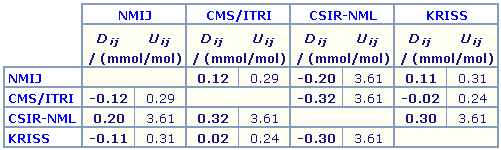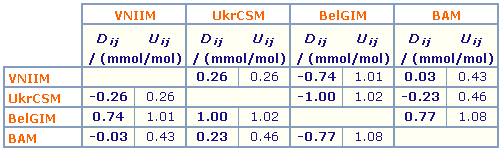All results, including relative degrees of equivalence for COOMET participants, are presented under A4 printable format in Summary Results (.PDF file).

CCQM-K3 was placed in the KCDB archives on 18 September 2023

CCQM-K3, EUROMET.QM-K3, APMP.QM-K3 and COOMET.QM-K3

MEASURAND : Amount-of-substance fraction of automotive emission gases

NOMINAL VALUES for Propane : ~ 2 mmol/mol

 Lab i Di Ui /(mmol/mol) /(mmol/mol) OMH -0.0006 0.0034 BAM 0.0091 0.0103 NIST 0.0006 0.0139 NMi-VSL 0.0000 0.0040 NMIJ 0.0000 0.0030 NRCCRM -0.0030 0.0071 SMU 0.0022 0.0301 KRISS 0.0013 0.0032 PTB -0.0078 0.0132 METAS -0.0159 0.0055 BNM-LNE -0.0030 0.0046 VNIIM -0.0077 0.0083 NPL 0.0050 0.0066 SMU 0.0044 0.0113 IMGC -0.0740 0.0720 IPQ 0.0002 0.0055 GUM -0.0006 0.0066 CMI 0.1219 0.1500 NMIJ -0.0026 0.0037 CMS/ITRI -0.0115 0.0171 KRISS 0.0021 0.0045 VNIIM 0.001 0.004 UkrCSM -0.001 0.004 BelGIM -0.004 0.020 BAM 0.010 0.012

In dark blue: laboratories participant in CCQM-K3
In green
: laboratories participant in EUROMET.QM-K3 (indicated as * in "Lab j" list)
In blue
: laboratories participant in APMP.QM-K3
In orange: laboratories participant in COOMET.QM-K3

Additional data: pair-wise degrees of equivalence inside APMP.QM-K3 and COOMET.QM-K3: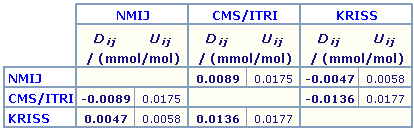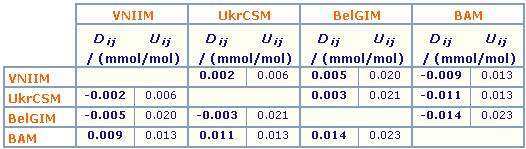All results, including relative degrees of equivalence for COOMET participants, are presented under A4 printable format in Summary Results (.PDF file).

Comparison
Comparison close
CC comparison
 Metrology area, Sub-field Chemistry and Biology, Gases Description Automotive emission gases Time of measurements 2000 Status Approved for equivalence
 Final Reports of the comparisons Measurand Amount-of-substance fraction of CO (32 mmol/mol), CO2 (135 mmol/mol) and C3H8 (2.05 mmol/mol); N2 balance Transfer device Calibrated gas cylinders measured by gravimetry
 Comparison type Key Comparison Consultative Committee CCQM (Consultative Committee for Amount of Substance) Conducted by EURAMET (European Association of National Metrology Institutes) RMO Internal Identifier EUROMET Project No 485 Comments EUROMET.QM-K3, APMP.QM-K3 and COOMET.QM-K3 results are linked to those of CCQM-K3. Dates of publication: CCQM-K3 results, 21 November 2001; EUROMET.QM-K3 results, 05 July 2002; APMP.QM-K3 results, 14 November 2003; COOMET.QM-K3 results, 31 August 2006. Automotive emission gases. Pilot institute VSL VSL Netherlands Contact person A. van der Veen +31 15 2691500
First Name Last Name
wwww@ww.www +356719836 Institute 1 Institute 1 Khmelnitskiy
Pilot laboratory
VSL

VSL, Netherlands, EURAMET

 CMI Czech Metrology Institute, Czechia, EURAMET
 GUM Glowny Urzad Miar, Central Office of Measures, Poland, EURAMET
 IPQ Instituto Portugues da Qualidade, Portugal, EURAMET
 SMU Slovensky Metrologicky Ustav, Slovakia, EURAMET
Comparison
Comparison close
Results

CCQM-K3 was placed in the KCDB archives on 18 September 2023

CCQM-K3, EUROMET.QM-K3, APMP.QM-K3 and COOMET.QM-K3

MEASURAND : Amount-of-substance fraction of automotive emission gases

NOMINAL VALUES for Carbon monoxide : 32 mmol/mol for CCQM-K3 and EUROMET.QM-K3, 28 mmol/mol for APMP.QM-K3, and 30 mmol/mol for COOMET.QM-K3

• Key comparison CCQM-K3

 Key comparison reference value: there is no single key comparison reference value for this comparison, the value xigrav is taken as the reference value for laboratory i.

 The degree of equivalence of each laboratory i with respect to the reference value is given by a pair of terms: Di = (xi - xigrav) and Ui, its expanded uncertainty (k = 2), both expressed in mmol/mol, Ui = 2(ui2 + uigravR2)1/2.

 The degree of equivalence between two laboratories i and j is given by a pair of terms: Dij =(Di - Dj) = (xi - xigrav) - (xj - xjgrav) and Uij, its expanded uncertainty (k = 2), both expressed in mmol/mol, Uij = 2(ui2 + uj2 + uigrav2 + ujgrav2)1/2.

• Key comparison EUROMET.QM-K3

 The gravimetric value xigrav-EUR is taken as the reference value for laboratory i participant in EUROMET.QM-K3.

 The degree of equivalence of laboratory i participant in EUROMET.QM-K3 with respect to the reference value is given by a pair of terms: Di-EUR = (xi-EUR - xigrav-EUR) and Ui-EUR, its expanded uncertainty (k = 2), both expressed in mmol/mol, Ui-EUR = 2(ui-EUR2 + uigravR-EUR2)1/2.

• Key comparison APMP.QM-K3

 The gravimetric value xigrav-APMP is taken as the reference value for laboratory i participant in APMP.QM-K3.

 The degree of equivalence of laboratory i participant in APMP.QM-K3 with respect to the reference value is given by a pair of terms: Di-APMP = (xi-APMP - xigrav-APMP) and Ui-APMP, its expanded uncertainty (k = 2), both expressed in mmol/mol, Ui-APMP = 2(ui-APMP2 + uigravR-APMP2)1/2.

• Key comparison COOMET.QM-K3

 The gravimetric value xigrav-COO is taken as the reference value for laboratory i participant in COOMET.QM-K3.

 The degree of equivalence of laboratory i participant in COOMET.QM-K3 with respect to the reference value is given by a pair of terms: Di-COO = (xi-COO - xigrav-COO) and Ui-COO, its expanded uncertainty (k = 2), both expressed in mmol/mol, Ui-COO = (ULab i-COO2 + UigravR-COO2)1/2 (since uncertainties are all expanded with k = 2). Relative values, expressed in %, are also calculated.

• Linking EUROMET.QM-K3, APMP.QM-K3, and COOMET.QM-K3 to CCQM-K3

 The regional QM-K3 key comparisons are linked to CCQM-K3 through the gravimetric values. It follows that:

 The degrees of equivalence with respect to the reference value obtained in the regional QM-K3 key comparisons are transferred to CCQM-K3 without correction: Di = Di-RMO and Ui = Ui-RMO, with "RMO" = "EUR" or "APMP" or "COO" according to the case.

 The degree of equivalence between two laboratories i and j, one and the other participant in any one of the four comparisons, is given by a pair of terms: Dij =(Di - Dj) and Uij, its expanded uncertainty (k = 2), both expressed in mmol/mol, Uij = 2(ui2 + uj2 + uigrav2 + ujgrav2)1/2, with indexes "-EUR", "R-APMP", and "R-COO" when necessary.

 These statements make it possible to extend the CCQM-K3 matrices of equivalence to participants in the regional QM-K3 key comparisons. In the following, not all pair-wise degrees of equivalence are explicitely computed.

CCQM-K3 was placed in the KCDB archives on 18 September 2023

CCQM-K3, EUROMET.QM-K3, APMP.QM-K3 and COOMET.QM-K3

MEASURAND : Amount-of-substance fraction of automotive emission gases

NOMINAL VALUES for Carbon dioxide : 135 mmol/mol for CCQM-K3, EUROMET.QM-K3 and COOMET.QM-K3, and 124 mmol/mol for APMP.QM-K3

• Key comparison CCQM-K3

 Key comparison reference value: there is no single key comparison reference value for this comparison, the value xigrav is taken as the reference value for laboratory i.

 The degree of equivalence of each laboratory i with respect to the reference value is given by a pair of terms: Di = (xi - xigrav) and Ui, its expanded uncertainty (k = 2), both expressed in mmol/mol, Ui = 2(ui2 + uigravR2)1/2.

 The degree of equivalence between two laboratories i and j is given by a pair of terms: Dij =(Di - Dj) = (xi - xigrav) - (xj - xjgrav) and Uij, its expanded uncertainty (k = 2), both expressed in mmol/mol, Uij = 2(ui2 + uj2 + uigrav2 + ujgrav2)1/2.

• Key comparison EUROMET.QM-K3

 The gravimetric value xigrav-EUR is taken as the reference value for laboratory i participant in EUROMET.QM-K3.

 The degree of equivalence of laboratory i participant in EUROMET.QM-K3 with respect to the reference value is given by a pair of terms: Di-EUR = (xi-EUR - xigrav-EUR) and Ui-EUR, its expanded uncertainty (k = 2), both expressed in mmol/mol, Ui-EUR = 2(ui-EUR2 + uigravR-EUR2)1/2.

• Key comparison APMP.QM-K3

 The gravimetric value xigrav-APMP is taken as the reference value for laboratory i participant in APMP.QM-K3.

 The degree of equivalence of laboratory i participant in APMP.QM-K3 with respect to the reference value is given by a pair of terms: Di-APMP = (xi-APMP - xigrav-APMP) and Ui-APMP, its expanded uncertainty (k = 2), both expressed in mmol/mol, Ui-APMP = 2(ui-APMP2 + uigravR-APMP2)1/2.

• Key comparison COOMET.QM-K3

 The gravimetric value xigrav-COO is taken as the reference value for laboratory i participant in COOMET.QM-K3.

 The degree of equivalence of laboratory i participant in COOMET.QM-K3 with respect to the reference value is given by a pair of terms: Di-COO = (xi-COO - xigrav-COO) and Ui-COO, its expanded uncertainty (k = 2), both expressed in mmol/mol, Ui-COO = (ULab i-COO2 + UigravR-COO2)1/2 (since uncertainties are all expanded with k = 2). Relative values, expressed in %, are also calculated.

• Linking EUROMET.QM-K3, APMP.QM-K3, and COOMET.QM-K3 to CCQM-K3

 The regional QM-K3 key comparisons are linked to CCQM-K3 through the gravimetric values. It follows that:

 The degrees of equivalence with respect to the reference value obtained in the regional QM-K3 key comparisons are transferred to CCQM-K3 without correction: Di = Di-RMO and Ui = Ui-RMO, with "RMO" = "EUR" or "APMP" or "COO" according to the case.

 The degree of equivalence between two laboratories i and j, one and the other participant in any one of the four comparisons, is given by a pair of terms: Dij =(Di - Dj) and Uij, its expanded uncertainty (k = 2), both expressed in mmol/mol, Uij = 2(ui2 + uj2 + uigrav2 + ujgrav2)1/2, with indexes "-EUR", "R-APMP", and "R-COO" when necessary.

 These statements make it possible to extend the CCQM-K3 matrices of equivalence to participants in the regional QM-K3 key comparisons. In the following, not all pair-wise degrees of equivalence are explicitely computed.

CCQM-K3 was placed in the KCDB archives on 18 September 2023

CCQM-K3, EUROMET.QM-K3, APMP.QM-K3 and COOMET.QM-K3

MEASURAND : Amount-of-substance fraction of automotive emission gases

NOMINAL VALUES for Propane : 2.05 mmol/mol for CCQM-K3 and EUROMET.QM-K3, 1.95 mmol/mol for APMP.QM-K3, and 2 mmol/mol for COOMET.QM-K3

• Key comparison CCQM-K3

 Key comparison reference value: there is no single key comparison reference value for this comparison, the value xigrav is taken as the reference value for laboratory i.

 The degree of equivalence of each laboratory i with respect to the reference value is given by a pair of terms: Di = (xi - xigrav) and Ui, its expanded uncertainty (k = 2), both expressed in mmol/mol, Ui = 2(ui2 + uigravR2)1/2.

 The degree of equivalence between two laboratories i and j is given by a pair of terms: Dij =(Di - Dj) = (xi - xigrav) - (xj - xjgrav) and Uij, its expanded uncertainty (k = 2), both expressed in mmol/mol, Uij = 2(ui2 + uj2 + uigrav2 + ujgrav2)1/2.

• Key comparison EUROMET.QM-K3

 The gravimetric value xigrav-EUR is taken as the reference value for laboratory i participant in EUROMET.QM-K3.

 The degree of equivalence of laboratory i participant in EUROMET.QM-K3 with respect to the reference value is given by a pair of terms: Di-EUR = (xi-EUR - xigrav-EUR) and Ui-EUR, its expanded uncertainty (k = 2), both expressed in mmol/mol, Ui-EUR = 2(ui-EUR2 + uigravR-EUR2)1/2.

• Key comparison APMP.QM-K3

 The gravimetric value xigrav-APMP is taken as the reference value for laboratory i participant in APMP.QM-K3.

 The degree of equivalence of laboratory i participant in APMP.QM-K3 with respect to the reference value is given by a pair of terms: Di-APMP = (xi-APMP - xigrav-APMP) and Ui-APMP, its expanded uncertainty (k = 2), both expressed in mmol/mol, Ui-APMP = 2(ui-APMP2 + uigravR-APMP2)1/2.

• Key comparison COOMET.QM-K3

 The gravimetric value xigrav-COO is taken as the reference value for laboratory i participant in COOMET.QM-K3.

 The degree of equivalence of laboratory i participant in COOMET.QM-K3 with respect to the reference value is given by a pair of terms: Di-COO = (xi-COO - xigrav-COO) and Ui-COO, its expanded uncertainty (k = 2), both expressed in mmol/mol, Ui-COO = (ULab i-COO2 + UigravR-COO2)1/2 (since uncertainties are all expanded with k = 2). Relative values, expressed in %, are also calculated.

• Linking EUROMET.QM-K3, APMP.QM-K3, and COOMET.QM-K3 to CCQM-K3

 The regional QM-K3 key comparisons are linked to CCQM-K3 through the gravimetric values. It follows that:

 The degrees of equivalence with respect to the reference value obtained in the regional QM-K3 key comparisons are transferred to CCQM-K3 without correction: Di = Di-RMO and Ui = Ui-RMO, with "RMO" = "EUR" or "APMP" or "COO" according to the case.

 The degree of equivalence between two laboratories i and j, one and the other participant in any one of the four comparisons, is given by a pair of terms: Dij =(Di - Dj) and Uij, its expanded uncertainty (k = 2), both expressed in mmol/mol, Uij = 2(ui2 + uj2 + uigrav2 + ujgrav2)1/2, with indexes "-EUR", "R-APMP", and "R-COO" when necessary.

 These statements make it possible to extend the CCQM-K3 matrices of equivalence to participants in the regional QM-K3 key comparisons. In the following, not all pair-wise degrees of equivalence are explicitely computed.

Comparison
Comparison close
Results

CCQM-K3 was placed in the KCDB archives on 18 September 2023

CCQM-K3, EUROMET.QM-K3, APMP.QM-K3 and COOMET.QM-K3

MEASURAND : Amount-of-substance fraction of automotive emission gases

• Key comparison CCQM-K3

 NOMINAL VALUES : Carbon monoxide 32 mmol/mol Carbon dioxide 135 mmol/mol Propane 2.05 mmol/mol Nitrogen Balance

xi : result of measurement carried out by laboratory i
ui : combined standard uncertainty of xi
xigrav : gravimetric value of the gas amount-of-substance fraction in the cylinder received by laboratory i
uigravR : combined standard uncertainty of xigrav

uigravR = (uigrav2 + uiver2)1/2, where uigrav and uiver are respectively the combined standard uncertainties of the gravimetric production process and of the analytical verification of the gravimetric value, with
uigrav = 0.0002 . xigrav and uiver = 0.0003 . xigrav, both in mmol/mol, for Carbon monoxide,
uigrav = 0.00003 . xigrav and uiver = 0.0003 . xigrav, both in mmol/mol, for Carbon dioxide, and
uigrav = 0.00012 . xigrav and uiver = 0.00056 . xigrav, both in mmol/mol, for Propane.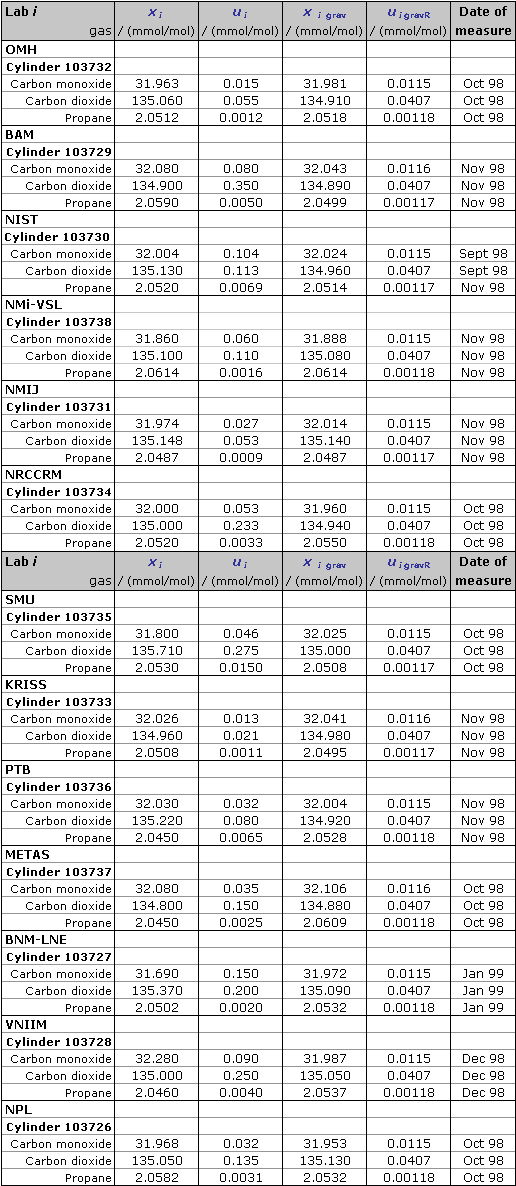• Key comparison EUROMET.QM-K3

 NOMINAL VALUES : Carbon monoxide 32 mmol/mol Carbon dioxide 135 mmol/mol Propane 2.05 mmol/mol Nitrogen Balance

xi-EUR : result of measurement carried out by laboratory i
ui-EUR : combined standard uncertainty of xi-EUR
xigrav-EUR : gravimetric value of the gas amount-of-substance fraction in the cylinder received by laboratory i
uigravR-EUR : combined standard uncertainty of xigrav-EUR

u igravR-EUR = (uigrav-EUR2 + uiver-EUR2)1/2, where uigrav-EUR and uiver-EUR are respectively the combined standard uncertainties of the gravimetric production process and of the analytical verification of the gravimetric value, with
uigrav-EUR = 0.0002 . xigrav-EUR and uiver-EUR = 0.0003 . xigrav-EUR , both in mmol/mol, for Carbon monoxide,
uigrav-EUR = 0.00003 . xigrav-EUR and uiver-EUR = 0.0003 . xigrav-EUR , both in mmol/mol, for Carbon dioxide, and
uigrav-EUR = 0.00012 . xigrav-EUR and uiver-EUR = 0.00056 . xigrav-EUR , both in mmol/mol, for Propane.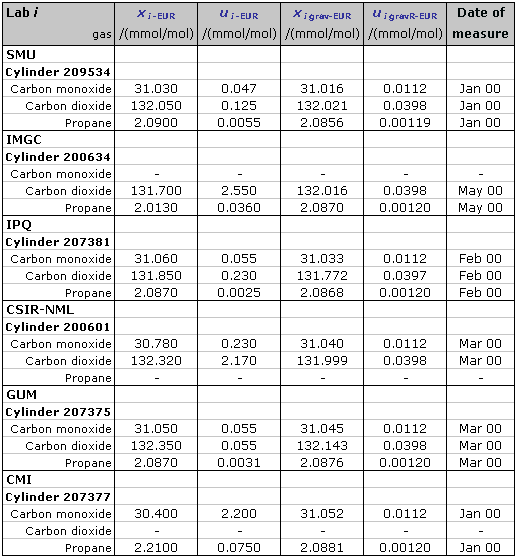• Key comparison APMP.QM-K3

 NOMINAL VALUES : Carbon monoxide 28 mmol/mol Carbon dioxide 124 mmol/mol Propane 1.95 mmol/mol Nitrogen Balance

xi-APMP : result of measurement carried out by laboratory i
ui-APMP : combined standard uncertainty of xi-APMP
xigrav-APMP : gravimetric value of the gas amount-of-substance fraction in the cylinder received by laboratory i
uigravR-APMP : combined standard uncertainty of xigrav-APMP

uigravR-APMP = (uigrav-APMP2 + uiver-APMP2)1/2, where uigrav-APMP and
uiver-APMP are respectively the combined standard uncertainties of the gravimetric production process and of the analytical verification of the gravimetric value.
The relative expanded uncertainty (k=2) linked to the verification process is estimated to be equal to 0.10%.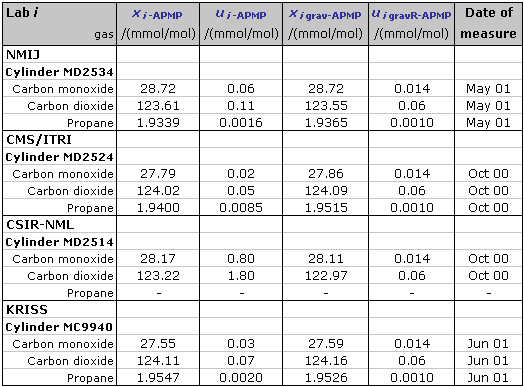• Key comparison COOMET.QM-K3

 NOMINAL VALUES : Carbon monoxide 30 mmol/mol Carbon dioxide 135 mmol/mol Propane 2 mmol/mol Nitrogen Balance

xi-COO : result of measurement carried out by laboratory i
ULab i-COO : expanded uncertainty (k = 2) of xi-COO
xigrav-COO : gravimetric value of the gas amount-of-substance fraction in the cylinder received by laboratory i
UigravR-COO : expanded uncertainty (k = 2) of xigrav-COO

UigravR-COO / 2 = (uigrav-COO2 + uiver-COO2)1/2, where uigrav-COO and
uiver-COO are respectively the combined standard uncertainties of the gravimetric production process and of the analytical verification of the gravimetric value.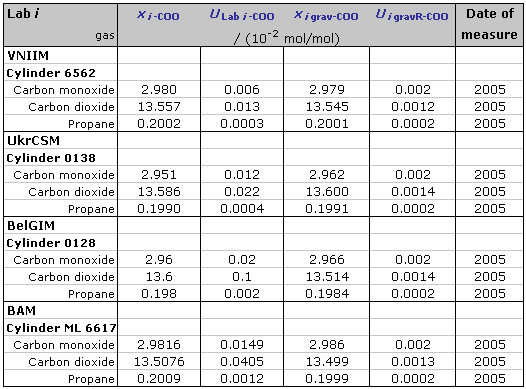CCQM-K3 was placed in the KCDB archives on 18 September 2023

CCQM-K3, EUROMET.QM-K3, APMP.QM-K3 and COOMET.QM-K3

MEASURAND : Amount-of-substance fraction of automotive emission gases

• Key comparison CCQM-K3

 NOMINAL VALUES : Carbon monoxide 32 mmol/mol Carbon dioxide 135 mmol/mol Propane 2.05 mmol/mol Nitrogen Balance

xi : result of measurement carried out by laboratory i
ui : combined standard uncertainty of xi
xigrav : gravimetric value of the gas amount-of-substance fraction in the cylinder received by laboratory i
uigravR : combined standard uncertainty of xigrav

uigravR = (uigrav2 + uiver2)1/2, where uigrav and uiver are respectively the combined standard uncertainties of the gravimetric production process and of the analytical verification of the gravimetric value, with
uigrav = 0.0002 . xigrav and uiver = 0.0003 . xigrav, both in mmol/mol, for Carbon monoxide,
uigrav = 0.00003 . xigrav and uiver = 0.0003 . xigrav, both in mmol/mol, for Carbon dioxide, and
uigrav = 0.00012 . xigrav and uiver = 0.00056 . xigrav, both in mmol/mol, for Propane.• Key comparison EUROMET.QM-K3

 NOMINAL VALUES : Carbon monoxide 32 mmol/mol Carbon dioxide 135 mmol/mol Propane 2.05 mmol/mol Nitrogen Balance

xi-EUR : result of measurement carried out by laboratory i
ui-EUR : combined standard uncertainty of xi-EUR
xigrav-EUR : gravimetric value of the gas amount-of-substance fraction in the cylinder received by laboratory i
uigravR-EUR : combined standard uncertainty of xigrav-EUR

u igravR-EUR = (uigrav-EUR2 + uiver-EUR2)1/2, where uigrav-EUR and uiver-EUR are respectively the combined standard uncertainties of the gravimetric production process and of the analytical verification of the gravimetric value, with
uigrav-EUR = 0.0002 . xigrav-EUR and uiver-EUR = 0.0003 . xigrav-EUR , both in mmol/mol, for Carbon monoxide,
uigrav-EUR = 0.00003 . xigrav-EUR and uiver-EUR = 0.0003 . xigrav-EUR , both in mmol/mol, for Carbon dioxide, and
uigrav-EUR = 0.00012 . xigrav-EUR and uiver-EUR = 0.00056 . xigrav-EUR , both in mmol/mol, for Propane.• Key comparison APMP.QM-K3

 NOMINAL VALUES : Carbon monoxide 28 mmol/mol Carbon dioxide 124 mmol/mol Propane 1.95 mmol/mol Nitrogen Balance

xi-APMP : result of measurement carried out by laboratory i
ui-APMP : combined standard uncertainty of xi-APMP
xigrav-APMP : gravimetric value of the gas amount-of-substance fraction in the cylinder received by laboratory i
uigravR-APMP : combined standard uncertainty of xigrav-APMP

uigravR-APMP = (uigrav-APMP2 + uiver-APMP2)1/2, where uigrav-APMP and
uiver-APMP are respectively the combined standard uncertainties of the gravimetric production process and of the analytical verification of the gravimetric value.
The relative expanded uncertainty (k=2) linked to the verification process is estimated to be equal to 0.10%.• Key comparison COOMET.QM-K3

 NOMINAL VALUES : Carbon monoxide 30 mmol/mol Carbon dioxide 135 mmol/mol Propane 2 mmol/mol Nitrogen Balance

xi-COO : result of measurement carried out by laboratory i
ULab i-COO : expanded uncertainty (k = 2) of xi-COO
xigrav-COO : gravimetric value of the gas amount-of-substance fraction in the cylinder received by laboratory i
UigravR-COO : expanded uncertainty (k = 2) of xigrav-COO

UigravR-COO / 2 = (uigrav-COO2 + uiver-COO2)1/2, where uigrav-COO and
uiver-COO are respectively the combined standard uncertainties of the gravimetric production process and of the analytical verification of the gravimetric value.CCQM-K3 was placed in the KCDB archives on 18 September 2023

CCQM-K3, EUROMET.QM-K3, APMP.QM-K3 and COOMET.QM-K3

MEASURAND : Amount-of-substance fraction of automotive emission gases

• Key comparison CCQM-K3

 NOMINAL VALUES : Carbon monoxide 32 mmol/mol Carbon dioxide 135 mmol/mol Propane 2.05 mmol/mol Nitrogen Balance

xi : result of measurement carried out by laboratory i
ui : combined standard uncertainty of xi
xigrav : gravimetric value of the gas amount-of-substance fraction in the cylinder received by laboratory i
uigravR : combined standard uncertainty of xigrav

uigravR = (uigrav2 + uiver2)1/2, where uigrav and uiver are respectively the combined standard uncertainties of the gravimetric production process and of the analytical verification of the gravimetric value, with
uigrav = 0.0002 . xigrav and uiver = 0.0003 . xigrav, both in mmol/mol, for Carbon monoxide,
uigrav = 0.00003 . xigrav and uiver = 0.0003 . xigrav, both in mmol/mol, for Carbon dioxide, and
uigrav = 0.00012 . xigrav and uiver = 0.00056 . xigrav, both in mmol/mol, for Propane.• Key comparison EUROMET.QM-K3

 NOMINAL VALUES : Carbon monoxide 32 mmol/mol Carbon dioxide 135 mmol/mol Propane 2.05 mmol/mol Nitrogen Balance

xi-EUR : result of measurement carried out by laboratory i
ui-EUR : combined standard uncertainty of xi-EUR
xigrav-EUR : gravimetric value of the gas amount-of-substance fraction in the cylinder received by laboratory i
uigravR-EUR : combined standard uncertainty of xigrav-EUR

u igravR-EUR = (uigrav-EUR2 + uiver-EUR2)1/2, where uigrav-EUR and uiver-EUR are respectively the combined standard uncertainties of the gravimetric production process and of the analytical verification of the gravimetric value, with
uigrav-EUR = 0.0002 . xigrav-EUR and uiver-EUR = 0.0003 . xigrav-EUR , both in mmol/mol, for Carbon monoxide,
uigrav-EUR = 0.00003 . xigrav-EUR and uiver-EUR = 0.0003 . xigrav-EUR , both in mmol/mol, for Carbon dioxide, and
uigrav-EUR = 0.00012 . xigrav-EUR and uiver-EUR = 0.00056 . xigrav-EUR , both in mmol/mol, for Propane.• Key comparison APMP.QM-K3

 NOMINAL VALUES : Carbon monoxide 28 mmol/mol Carbon dioxide 124 mmol/mol Propane 1.95 mmol/mol Nitrogen Balance

xi-APMP : result of measurement carried out by laboratory i
ui-APMP : combined standard uncertainty of xi-APMP
xigrav-APMP : gravimetric value of the gas amount-of-substance fraction in the cylinder received by laboratory i
uigravR-APMP : combined standard uncertainty of xigrav-APMP

uigravR-APMP = (uigrav-APMP2 + uiver-APMP2)1/2, where uigrav-APMP and
uiver-APMP are respectively the combined standard uncertainties of the gravimetric production process and of the analytical verification of the gravimetric value.
The relative expanded uncertainty (k=2) linked to the verification process is estimated to be equal to 0.10%.• Key comparison COOMET.QM-K3

 NOMINAL VALUES : Carbon monoxide 30 mmol/mol Carbon dioxide 135 mmol/mol Propane 2 mmol/mol Nitrogen Balance

xi-COO : result of measurement carried out by laboratory i
ULab i-COO : expanded uncertainty (k = 2) of xi-COO
xigrav-COO : gravimetric value of the gas amount-of-substance fraction in the cylinder received by laboratory i
UigravR-COO : expanded uncertainty (k = 2) of xigrav-COO

UigravR-COO / 2 = (uigrav-COO2 + uiver-COO2)1/2, where uigrav-COO and
uiver-COO are respectively the combined standard uncertainties of the gravimetric production process and of the analytical verification of the gravimetric value.